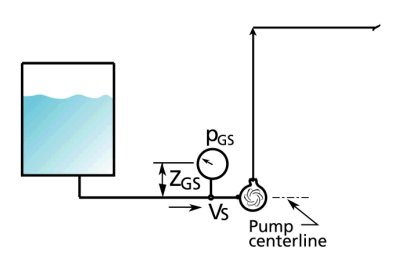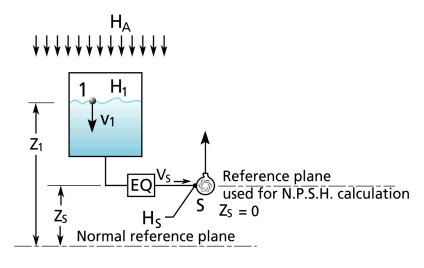# HELP FOR THE N.P.S.H.A. APPLET PROGRAM

This document will cover two topics, one a general discussion of this subject and how the equations were developped. The other some specific comments on how the applet functions.

General

N.P.S.H.A. means Net Positive Suction Head Available. It is a term used in the pump industry and it indicates whether there is enough pressure at the pump suction for the pump to operate properly. If there is insufficient pressure the pump will not operate as expected and the flow will be reduced and damage will occur. If the liquid has insufficient pressure energy at the suction this means that it is difficult to get the fluid into the impeller eye and into the impeller where the pressurization starts and the work of the pump is accomplished. This does not mean that the pump cannot operate even if the suction pressure is low, the pressure can be below atmospheric pressure (for example below 0 psig) and still operate satisfactorily. It is the value of N.P.S.H.A., which includes pressure level and other factors, that will determine whether the pump will operate properly or not.

The N.P.S.H.A. is compared to the N.P.S.H.R. (Net Positive Suction Head Required) which is given by the manufacturer for a specific pump at a specific speed and flow rate. The value of N.P.S.H.A. must always be higher than the vales of N.P.S.H.R.

N.P.S.H.A. is a head term and is expressed in feet in the Imperial system and meters in the metric system. Head can be converted to pressure as is explained in the help document of the head to pressure conversion applet.

The N.P.S.H.A. also depends on the vapor pressure of the fluid. The vapor pressure of a fluid is the pressure at which a fluid boils for a given temperature. For example, water boils at 0 psia when the temperature is 212 F. Water can also boil at 1.69 psia when the temperature is 120 F. This means that fluids can boil at lower temperatures when the pressure is reduced. This experiment on this video shows that it is possible to boil water at room temperature if the pressure is low enough.

When a fluid enters the pump suction, the pressure drops considerably near the eye of the impeller, if the pressure goes below the vapor pressure of the liquid then the liquid will boil and a mixture of vapor and liquid enters the pump reducing its capacity. Cavitation will result creating harmful erosion to the pump impeller. You can hear the sound of cavitation on this audio recording. It is a very characteristic sound which resembles gravel in a concrete mixer.

This picture shows the damage to the impeller that can result from cavitation.To avoid this, in the definition of the N.P.S.H.A., the pressure at the pump suction is subtracted from the vapor pressure head so that the value of N.P.S.H.A. will always represents a value that is superior to the vapor pressure head of the liquid.

If you know the pressure at the pump inlet, then the value for N.P.S.H.A. is:SG is the specific gravity of the liquid, for water at room temperature SG=1.0, and g is the acceleration due to gravity constant and is worth 32.17 ft/s2.

The following figure will make the location of the pressure measurement clear. In order to make all measurements of N.P.S.H.A. comparable it is necessary to relate all pressure measurements to one reference, the pump suction centerline. The pressure gauge reading once converted to head will have to be added from the height of the gauge with respect to the pump suction centerline. This is what the terms zGS is for. pA is the atmospheric pressure in the local environment and depends on elevation. If the pump is close to sea level then pA is 14.7 psia. pva is the vapor pressure of the liquid and depends on temperature, you can find values for vapor pressure here. pGS is the pressure measurement at the pump suction in psig, and vS is the velocity on the liquid in the suction line and depends on the flow rate ( see the help page of the pressure to head applet).If a pressure gauge is not available on the pump suction, N.P.S.H.A. can be calculated.More terms are required to do this calculation because the pressure at the pump suction is the result of pressure losses and equipment losses in the suction line, if there is no pressure measurement then the losses must be calculated. This is what terms HF1-S and HEQ1-S are for, they are respectively the pressure head losses for the pipe and fittings and the equipment in the suction line. The other terms are self-explanatory and can be seen in the following figure.To summarize, the N.P.S.H.A. can be easily calculated if the pressure is known at the pump suction. If the pressure is unknown, then the N.P.S.H.A. can be calculated based on the head losses incurred in the suction line.

This document will cover two topics, one a general discussion of this subject and how the equations were developped. The other some specific comments on how the applet functions.

Applets are programs based on the java language that are designed to run on your computer using the Java Run Time environment.

This program is a simple calculation which leaves most of the details such as, calculating fluid friction loss between the fluid surface and the pump suction, finding the vapor pressure of your fluid, and finding the value for the local atmospheric pressure, up to you. What it does give you is the correct formula for estimating the N.P.S.H.A. (Net Positive Suction head Available) once you have all the data. There are two separate methods used to calculate N.P.S.H.A.. The first one is based on the fluid surface height and the second on a pressure measurement taken at the pump suction.

N.P.S.H.A. knowing the tank level

This is additional information you need to know about the data that you can input into this part of the program:

z: pump suction static head or the difference in elevation between the tank liquid surface and the pump suction, you can enter a positive or negative number;

H1: the pressure on the fluid surface of the tank, if the tank is open to atmosphere then this is zero, if the tank is pressurized then this could be anything, positive or negative;

Hf: the friction head loss between the tank fluid surface and the pump suction, normally this is the loss in the suction pipe, you can enter only a positive number;

Ha: the value of the local atmospheric pressure head, at sea level the pressure is 14.7 psia, this corresponds to 34 feet of water absolute which is the value you would enter in the textbox, only positive numbers can be entered;

Hva: the value of the fluid vapor pressure head, water has a vapor pressure of 0.5 psia which corresponds to a pressure head of 0.6 feet of water absolute.

The small tank in the applet will change as the z value changes giving you the position of the fluid surface vs. the pump suction. As you can see, the pump suction has an elbow on the end of the pipe. Don't worry too much about this if your installation does not have such an elbow this will add only a small amount of friction loss.

The friction loss Hf you will have to estimate, however consider that you typically should not have a higher value than 1 foot of pressure head loss to be safe. The fluid surface tank pressure H1 is the pressure value in terms of pressure head that corresponds to the pressure in the tank if any. If this is an open tank then H1 =0 feet of pressure head. If you have pressure say of 5 psig then the pressure head that corresponds is 5 * 2.31/SG. SG is the specific gravity of the fluid.

N.P.S.H.A. knowing the pressure at the pump suction

This is additional information you need to know about the data that you can input into this part of the program:

Hs: pump suction pressure head, this is the measured pressure at the pump suction converted to pressure head, you can enter enter a positive or negative number;

Hvs: the velocity head at the pump suction, knowing the flow rate you can calculated the velocity in ft/s and from the velocity calculate the velocity head in feet (=v^2/2 /32.17), you can enter enter a positive or negative number;

zg: the height in feet of the pressure gauge above the pump centerline, you can enter enter a positive or negative number;

The default value for Hvs is set at 0.75 feet, this is a conservative estimate. Consider that a typical suction velocity should not exceed 5 ft/s which corresponds to a velocity head of 0.4 feet.

ENJOY!